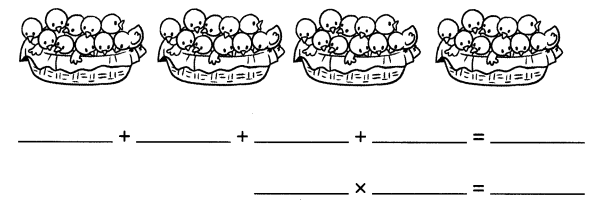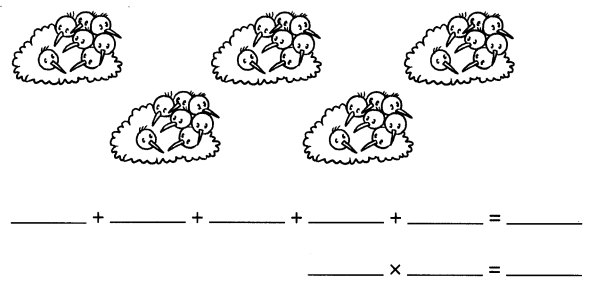# Math in Focus Grade 2 Chapter 5 Practice 1 Answer Key How to Multiply

Go through the Math in Focus Grade 2 Workbook Answer Key Chapter 5 Practice 1 How to Multiply to finish your assignments.

## Math in Focus Grade 2 Chapter 5 Practice 1 Answer Key How to Multiply

Look at the pictures.
Fill in the blanks.

Example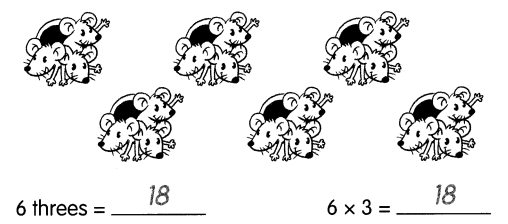Question 1.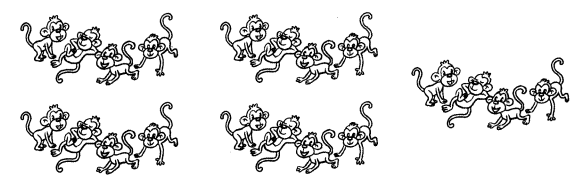5 groups of 4 monkeys = ___
5 × 4 = ___
Given that
Number of groups are 5
Number of monkeys in each group are 4
5 groups of 4 monkeys are 5 × 4 = 20.

Count and add the number of animals in each group. Then multiply.

Example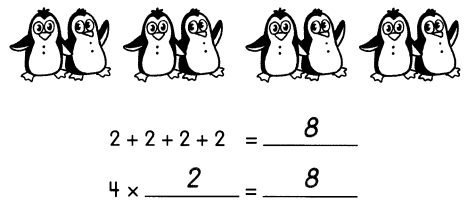Question 2.